Chapter 6: Polynomials

# 6.8 Mixture and Solution Word Problems

Solving mixture problems generally involves solving systems of equations. Mixture problems are ones in which two different solutions are mixed together, resulting in a new, final solution. Using a table will help to set up and solve these problems.  The basic structure of this table is shown below:

Example Mixture Problem Solution Table
Name Amount Value Equation

The first column in the table (Name) is used to identify the fluids or objects being mixed in the problem. The second column (Amount) identifies the amounts of each of the fluids or objects. The third column (Value) is used for the value of each object or the percentage of concentration of each fluid. The last column (Equation) contains the product of the Amount times the Value or Concentration.

Example 6.8.1

Jasnah has 70 mL of a 50% methane solution. How much of a 80% solution must she add so the final solution is 60% methane? Find the equation.

• The solution names are 50% (S50), 60% (S60), and 80% (S80).
• The amounts are S50 = 70 mL, S80, and S60 = 70 mL + S80.
• The concentrations are S50 = 0.50, S60 = 0.60, and S80 = 0.80.
Name Amount Value Equation
S50 70 mL 0.50 0.50 (70 mL)
S80 S80 0.80 0.80 (S80)
S60 70 mL + S80 0.60 0.60 (70 mL + S80)

The equation derived from this data is 0.50 (70 mL) + 0.80 (S80) = 0.60 (70 mL + S80).

Example 6.8.2

Sally and Terry blended a coffee mix that sells for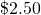by mixing two types of coffee. If they used 40 mL of a coffee that costs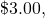how much of another coffee costing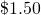did they mix with the first?

Name Amount Value Equation
C1.50 C1.50(C1.50)
C3.00 40 mL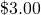(40 mL)
C2.50 40 mL + C1.50(40 mL + C1.50)

The equation derived from this data is: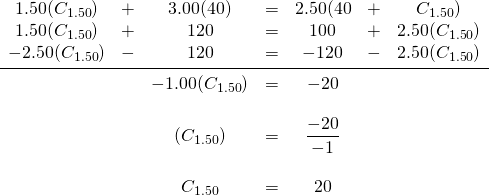This means 20 mL of the coffee selling foris needed for the mix.

Example 6.8.3

Nick and Chloe have two grades of milk from their small dairy herd: one that is 24% butterfat and another that is 18% butterfat. How much of each should they use to end up with 42 litres of 20% butterfat?

Name Amount Value Equation
B24 B24 0.24 0.24 (B24)
B18 42 L − B24 0.18 0.18 (42 L − B24)
B20 42 L 0.20 0.20 (42 L)

The equation derived from this data is: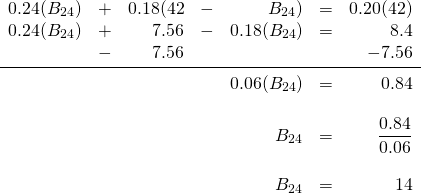This means 14 litres of the 24% buttermilk, and 28 litres of the 18% buttermilk is needed.

Example 6.8.4

In Natasha’s candy shop, chocolate, which sells for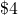a kilogram, is mixed with nuts, which are sold fora kilogram. Chocolate and nuts are combined to form a chocolate-nut candy, which sells for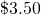a kilogram. How much of each are used to make 30 kilograms of the mixture?

Name Amount Value Equation
Chocolate C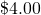(C)
Nuts 30 kg − C(30 kg − C)
Mix 30 kg(30 kg)

The equation derived from this data is: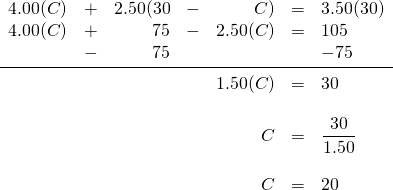Therefore, 20 kg of chocolate is needed for the mixture.

With mixture problems, there is often mixing with a pure solution or using water, which contains none of the chemical  of interest. For pure solutions, the concentration is 100%. For water, the concentration is 0%. This is shown in the following example.

Example 6.8.5

Joey is making a a 65% antifreeze solution using pure antifreeze mixed with water. How much of each should be used to make 70 litres?

Name Amount Value Equation
Antifreeze (A) A 1.00 1.00 (A)
Water (W) 70 L − A 0.00 0.00 (70 L − A)
65% Solution 70 L 0.65 0.65 (70 L)

The equation derived from this data is: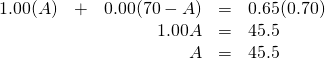This means the amount of water added is 70 L − 45.5 L = 24.5 L.

# Questions

For questions 1 to 9, write the equations that define the relationship.

1. A tank contains 8000 litres of a solution that is 40% acid. How much water should be added to make a solution that is 30% acid?
2. How much pure antifreeze should be added to 5 litres of a 30% mixture of antifreeze to make a solution that is 50% antifreeze?
3. You have 12 kilograms of 10% saline solution and another solution of 3% strength. How many kilograms of the second should be added to the first in order to get a 5% solution?
4. How much pure alcohol must be added to 24 litres of a 14% solution of alcohol in order to produce a 20% solution?
5. How many litres of a blue dye that costs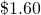per litre must be mixed with 18 litres of magenta dye that costsper litre to make a mixture that costs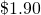per litre?
6. How many grams of pure acid must be added to 40 grams of a 20% acid solution to make a solution which is 36% acid?
7. A 100-kg bag of animal feed is 40% oats. How many kilograms of pure oats must be added to this feed to produce a blend of 50% oats?
8. A 20-gram alloy of platinum that costs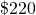per gram is mixed with an alloy that costs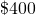per gram. How many grams of thealloy should be used to make an alloy that costs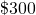per gram?
9. How many kilograms of tea that costper kilogram must be mixed with 12 kilograms of tea that cost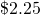per kilogram to make a mixture that costs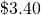per kilogram?

Solve questions 10 to 21.

1. How many litres of a solvent that costs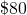per litre must be mixed with 6 litres of a solvent that costs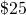per litre to make a solvent that costs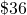per litre?
2. How many kilograms of hard candy that cost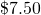per kg must be mixed with 24 kg of jelly beans that cost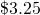per kg to make a mixture that sells for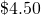per kg?
3. How many kilograms of soil supplement that costs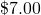per kg must be mixed with 20 kg of aluminum nitrate that costsper kg to make a fertilizer that costsper kg?
4. A candy mix sells for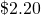per kg. It contains chocolates worthper kg and other candy worthper kg. How much of each are in 15 kg of the mixture?
5. A certain grade of milk contains 10% butterfat and a certain grade of cream 60% butterfat. How many litres of each must be taken so as to obtain a mixture of 100 litres that will be 45% butterfat?
6. Solution A is 50% acid and solution B is 80% acid. How much of each should be used to make 100 cc of a solution that is 68% acid?
7. A paint that contains 21% green dye is mixed with a paint that contains 15% green dye. How many litres of each must be used to make 600 litres of paint that is 19% green dye?
8. How many kilograms of coffee that is 40% java beans must be mixed with coffee that is 30% java beans to make an 80-kg coffee blend that is 32% java beans?
9. A caterer needs to make a slightly alcoholic fruit punch that has a strength of 6% alcohol. How many litres of fruit juice must be added to 3.75 litres of 40% alcohol?
10. A mechanic needs to dilute a 70% antifreeze solution to make 20 litres of 18% strength. How many litres of water must be added?
11. How many millilitres of water must be added to 50 millilitres of 100% acid to make a 40% solution?
12. How many litres of water need to be evaporated from 50 litres of a 12% salt solution to produce a 15% salt solution?